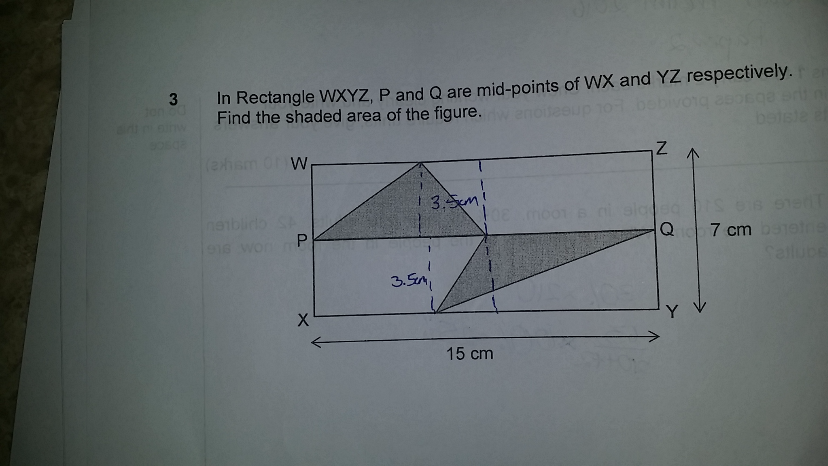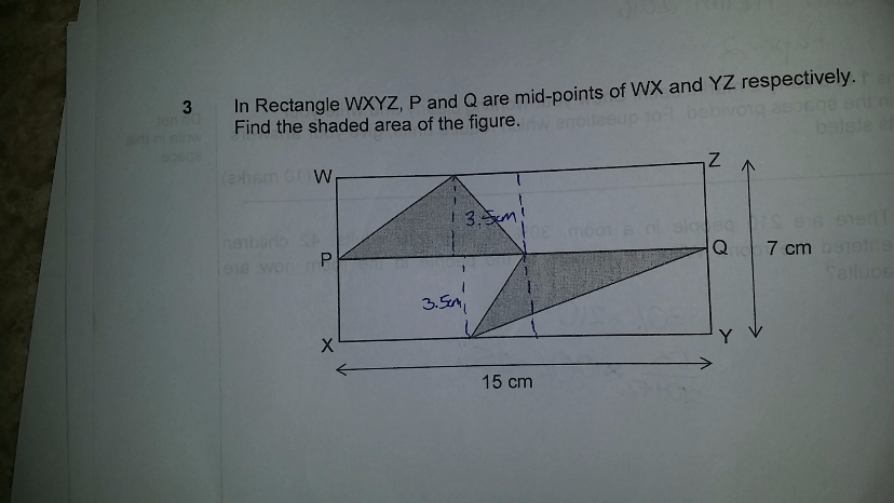# QuestionAppreciate your help to solve this question from Tao Nan Prelim 2016 paper. DS said we cannot assume the base is equal.

Thanks

Source: Tao Nanarea of top triangle +area of bottom triangle = 1/2 × top base  x 3.5 + 1/2 × bottom base×3.5

=1/2 × 3.5 × (top base + bottom base)

=1/2 ×3.5× 15 = 105/4

note: yr ds is correct. Cannot assume it is equal base. But if the height is the same, we can add the 2 bases which is equal to 14.

Thanks Piggylalala and Chief!

Will get DS to memorize this concept.

0 Replies 0 Likes

A useful method I called it the “rubber band” vertex rule, where the vertex of triangle moves horizontally along the parallel line (height) above the base and the base of triangle remains the same, area of triangle remains the same. (A key concept)

If we flip the bottom shaded triangle up, the height of both riangles are the same with the vertices along the top line. Joining both vertices on any point along that line will give a combined triangle in the TOP half the rectangle (Base of triangle is PQ).

Area is 1/2 x 15 x 3.5 = 26.25 sq cm#

0 Replies 0 Likes